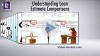# APR, TIP and Cost-Over-Time Comparisons In Loan EstimatesThe Loan Estimate form helps you compare different loans; Page 3 of the form summarizes the key figures.

• Cost-over-time figures (“In ___ Years”) chart out the total amount paid, and the amount applied against loan principal.  This ratio usually changes over time.
• Annual Percentage Rate – APR – gives you the ratio of interest PLUS any applicable fees for a complete percentage cost, per year.
• Total Interest Percentage – TIP – shows interest over the life of the loan, in relation to the loan amount.  For example, a \$500K loan with a TIP of 20% indicates that you would pay \$600K (20% of \$500K = \$100K) in total.

### You might also like